mraa  2.0.0 Low Level Skeleton Library for Communication on GNU/Linux platforms
Spi Class Reference

## Detailed Description

This file defines the SPI interface for libmraa

mraa::Spi spi(SPI_PORT);
while (flag) {
for (i = 90; i < 130; i++) {
data = i;
recv = spi.write(data, 2);
std::cout << "Writing -%i" << i << std::endl;
if (recv) {
std::cout << "RECIVED-%i-%i" << recv << recv << std::endl;
free(recv);
}
usleep(100000);
}
for (i = 130; i > 90; i--) {
data = i;
if (spi.transfer(data, rxBuf, 2) == mraa::SUCCESS) {
std::cout << "Writing -%i" << i << std::endl;
std::cout << "RECIVED-%i-%i" << rxBuf << rxBuf << std::endl;
}
usleep(100000);
}
}

## Public Member Functions

Spi (int bus)

Spi (int bus, int cs)

Spi (void *spi_context)

~Spi ()

Result mode (Spi_Mode mode)

Result frequency (int hz)

int writeByte (uint8_t data)

int writeWord (uint16_t data)

uint8_t * write (uint8_t *txBuf, int length)

uint16_t * writeWord (uint16_t *txBuf, int length)

Result transfer (uint8_t *txBuf, uint8_t *rxBuf, int length)

Result transfer_word (uint16_t *txBuf, uint16_t *rxBuf, int length)

Result lsbmode (bool lsb)

Result bitPerWord (unsigned int bits)

## Constructor & Destructor Documentation

 Spi ( int bus )
inline

Initialise SPI object using the board mapping to set muxes

Parameters
 bus to use, as listed in the platform definition, normally 0

Here is the call graph for this function: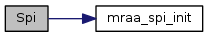Spi ( int bus, int cs )
inline

Initialise SPI object using 'raw' mode. Mraa will go and grab the spidev device lablled /dev/spidev[bus].[cs]

Parameters
 bus to use cs to use

Here is the call graph for this function: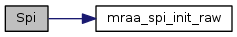Spi ( void * spi_context )
inline

Spi Constructor, takes a pointer to a SPI context and initialises the SPI class

Parameters
 spi_context void * to SPI context
 ~Spi ( )
inline

Closes spi bus

Here is the call graph for this function: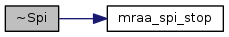## Member Function Documentation

 Result mode ( Spi_Mode mode )
inline

Set the SPI device mode. see spidev0-3

Parameters
 mode the mode. See Linux spidev doc
Returns
Result of operation

Here is the call graph for this function: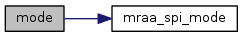Result frequency ( int hz )
inline

Set the SPI device operating clock frequency

Parameters
 hz the frequency to set in hz
Returns
Result of operation

Here is the call graph for this function: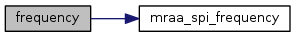int writeByte ( uint8_t data )
inline

Write single byte to the SPI device

Parameters
 data the byte to send
Returns
data received on the miso line or -1 in case of error

Here is the call graph for this function: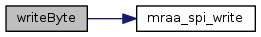int writeWord ( uint16_t data )
inline

Write single byte to the SPI device

Parameters
 data the byte to send
Returns
data received on the miso line or -1 in case of error

Here is the call graph for this function: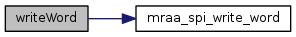uint8_t* write ( uint8_t * txBuf, int length )
inline

Write buffer of bytes to SPI device The pointer return has to be free'd by the caller. It will return a NULL pointer in cases of error

Parameters
 txBuf buffer to send length size of buffer to send
Returns
uint8_t* data received on the miso line. Same length as passed in

Here is the call graph for this function: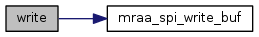uint16_t* writeWord ( uint16_t * txBuf, int length )
inline

Write buffer of bytes to SPI device The pointer return has to be free'd by the caller. It will return a NULL pointer in cases of error

Parameters
 txBuf buffer to send length size of buffer (in bytes) to send
Returns
uint8_t* data received on the miso line. Same length as passed in

Here is the call graph for this function:Result transfer ( uint8_t * txBuf, uint8_t * rxBuf, int length )
inline

Transfer data to and from SPI device Receive pointer may be null if return data is not needed.

Parameters
 txBuf buffer to send rxBuf buffer to optionally receive data from spi device length size of buffer to send
Returns
Result of operation

Here is the call graph for this function: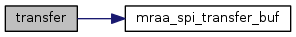Result transfer_word ( uint16_t * txBuf, uint16_t * rxBuf, int length )
inline

Transfer data to and from SPI device Receive pointer may be null if return data is not needed.

Parameters
 txBuf buffer to send rxBuf buffer to optionally receive data from spi device length size of buffer to send
Returns
Result of operation

Here is the call graph for this function: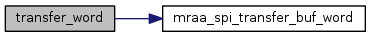Result lsbmode ( bool lsb )
inline

Change the SPI lsb mode

Parameters
 lsb Use least significant bit transmission - 0 for msbi
Returns
Result of operation

Here is the call graph for this function:Result bitPerWord ( unsigned int bits )
inline

Set bits per mode on transaction, default is 8

Parameters
 bits bits per word
Returns
Result of operation

Here is the call graph for this function:The documentation for this class was generated from the following file: